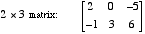index: click on a letter A B C D E F G H I J K L M N O P Q R S T U V W X Y Z A to Z index index: subject areas numbers & symbols sets, logic, proofs geometry algebra trigonometry advanced algebra & pre-calculus calculus advanced topics probability & statistics real world applications multimedia entrieswww.mathwords.com about mathwords website feedback

 Dimensions of a Matrix The number of rows and columns of a matrix, written in the form rows×columns. The matrix below has 2 rows and 3 columns, so its dimensions are 2×3. This is read aloud, "two by three." Note: One way to remember that Rows come first and Columns come second is by thinking of RC Cola®.See also Square matrix